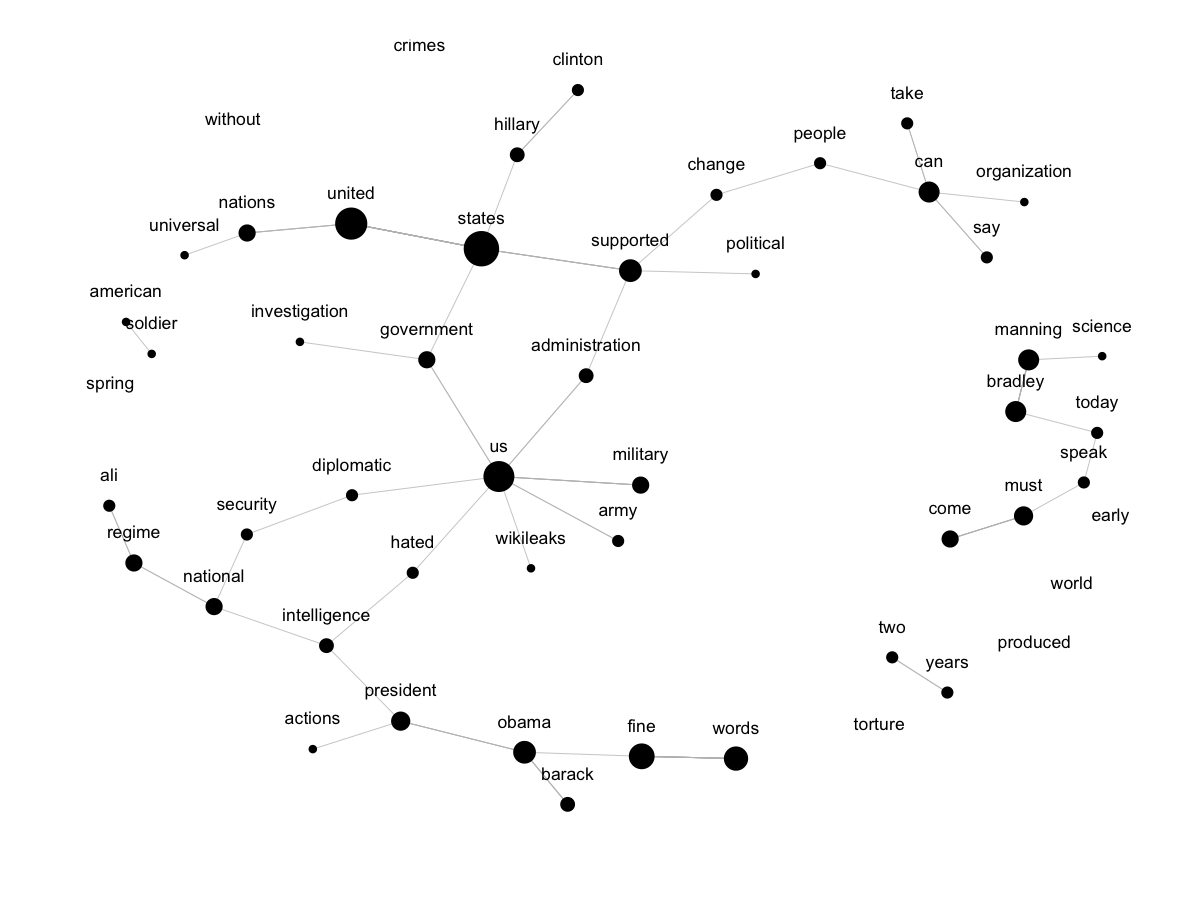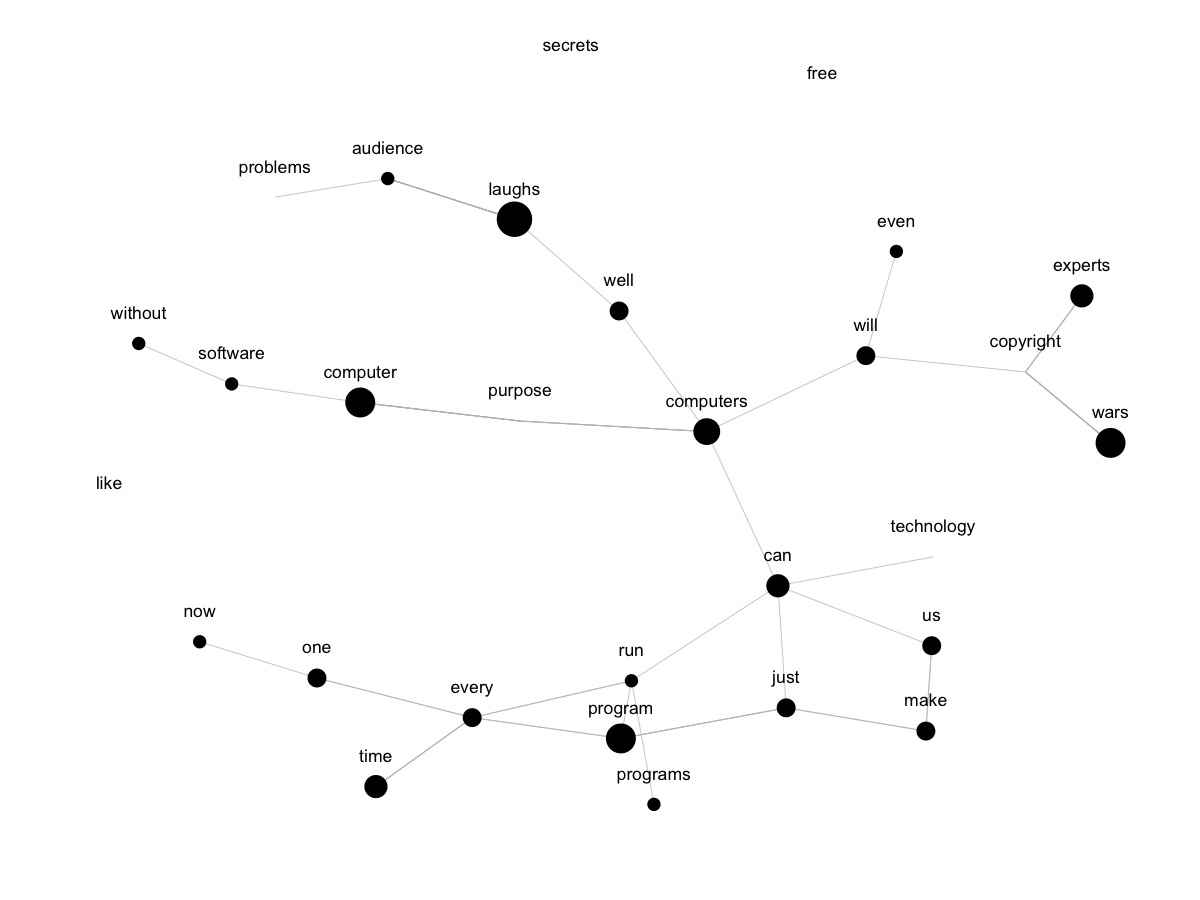Introduction to Data Analysis

# 11.2. Network(d)s

This section brings a bit of text mining into network analysis, as we will turn word associations into network ties (networks of word associations) and visualize the result. The code draws on the ideas of Cornelius Puschmann, and the example data is Julian Assange's address at the United Nations in September 2012.

packages <- c("intergraph", "GGally", "ggplot2", "network", "RColorBrewer",
"sna", "tm")
packages <- lapply(packages, FUN = function(x) {
if (!require(x, character.only = TRUE)) {
install.packages(x)
library(x, character.only = TRUE)
}
})


Our first step is to read the text file, to extract all words from the speech, and to extract all associations like “welcome to” or “fine deeds”. We replace Cornelius Puschmann's original code by a functional approach to the job, using one the many R apply functions (sapply being from the plyr package) for the main word association routine.

build.corpus <- function(x, skip = 0) {
src = scan(x, what = "char", sep = "\n", encoding = "UTF-8", skip = skip)
# Extract all words.
txt = unlist(strsplit(gsub("[[:punct:]|[:digit:]]", " ", tolower(src)),
"[[:space:]]+"))
# Remove single letters.
txt = txt[nchar(txt) > 1]
# Function to create word nodes.
associate <- function(x) {
y = c(txt[x], txt[x + 1])
if (!TRUE %in% (y %in% c("", stopwords("en"))))
y
}
# Build word network.
net = do.call(rbind, sapply(1:(length(txt) - 1), associate))
# Return network object.
return(network(net))
}
# Example data.
net <- build.corpus("data/assange.txt")


The word network is plotted as a very sparse network, trimmed to associations of non-trivial words that appear at least three times in the speech. Trivial words are removed by matching them to the list of English stopwords found in the tm package. We again use the ggnet function, but have a look at the igraph package for an alternative.

# Plot with ggnet.
ggnet(net, weight = "degree", subset = 3,
alpha = 1, segment.color = "grey", label = TRUE, vjust = - 2,
legend = "none")

52 nodes, weighted by freeman

id indegree outdegree freeman
39 states       11         6      17
45 united        0        14      14
47     us        1        12      13
15   fine        1         8       9
50  words        8         0       8
26  obama        6         1       7Since the corpus was created out of a simple function call, we can now find any corpus, prepare it and plot it in just a few lines. The next example is a plot of word associations in Cory Doctorow's speech to the Chaos Communication Congress in December 2011. Try running the same graph on any plain text speech file (here's one by Barack Obama).

# Target locations
file = "data/doctorow.txt"
# Build corpus.
net <- build.corpus(file, skip = 37)
# Plot with ggnet.
ggnet(net, weight = "indegree", subset = 5,
alpha = 1, segment.color = "grey", label = TRUE, vjust = - 2,
legend = "none")

29 nodes, weighted by indegree

id indegree outdegree freeman
11    laughs        7         1       8
3   computer        5         1       6
17   program        5         1       6
26      wars        5         0       5
4  computers        4         3       7
2        can        3         2       5